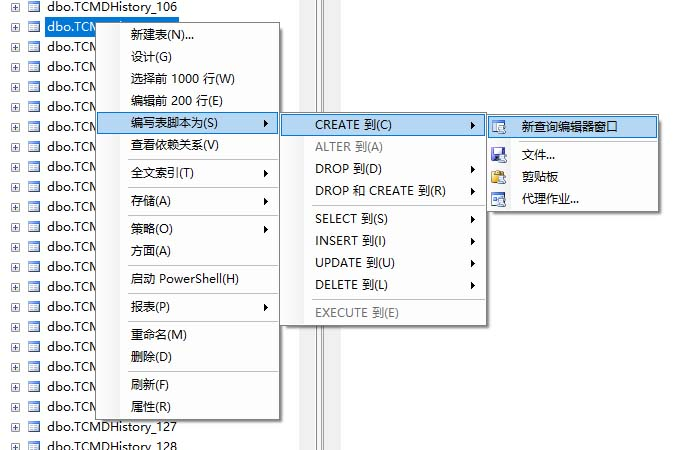2017-12-09 07:14

# 这样的存储过程怎么写啊，求大神指点（SQL SERVER）

20
• 数据库

``````SET ANSI_NULLS ON
GO
SET QUOTED_IDENTIFIER ON
GO
ALTER PROCEDURE [dbo].[bb_sx_zptj]
@KSRQ varchar(20),      --开始时间
@JSRQ varchar(20)      --结束时间
AS
BEGIN

drop table t_zptj      --这边删除，因为要根据每次输入的时间来重新进行数据统计
create table t_zptj(
月份       datetime,
装配总数      varchar(20),
)

--2.装配总数
update t_zptj 装配总数=temp1.发货数量 from
(select SUM(1) as 发货数量 from T_CONTRACT_ORDER a left join T_CONTRACT_ORDER_detail b
on a.sub_account_id=b.sub_account_id
where a.EXTEND50>=@KSRQ and a.extend50<=@JSRQ and a.EXTEND1='装配工程单'
group by b.OUT_NUM)
temp1
where t_zptj.装配总数=temp1.发货数量

``````

T_CONTRACT_ORDER (订单表)
T_CONTRACT_ORDER_detail （订单明细表） //这是要查询的2张表

1） 2表的sub_account_id字段（即订单的所有人）相等
2） extend1 (订单表)
T_CONTRACT_ORDER .extend1='装配工程单' --订单的分类条件，表明是我需要的装配类订单。

``````        3）    out_num（订单明细表）
T_CONTRACT_ORDER_detail .out_num   装配数量

4）  extend50(订单表)
T_CONTRACT_ORDER .extend50     订单的时间
``````

create table t_zptj(

)

``````        根据输入的时间参数和订单时间进行比较

2014年3月  结束时间
``````

2017-2 240
2017-3 120

• 点赞
• 写回答
• 关注问题
• 收藏
• 复制链接分享
• 邀请回答

#### 6条回答

• 你的问题没有描述清楚，你应该描述你有哪两张表，参与计算的有什么字段，需要得到什么结果，结果对应条件，否则没法解答

点赞 1 评论 复制链接分享
• 我看到前面还有一题是不是也是你发的，这个是你要做的一套结构么？你现在相当于是把统计数据放到一张临时表里，然后显示出来对吧，那不应该用这个方案来处理，另外你这个装配总数是在订单表还是在订单明细表里的？如果你的订单明细不需要统计数据可以不用left join处理,针对这个你可以用两个不同的存储过程来处理，一个是只统计订单上数据的，另一个是统计订单和明细数据的。
end语法错误那里你可以鼠标移动到那个错误上可以看到具体错误的解释，有可能是整个语句缺少什么东西了.

点赞 评论 复制链接分享
• 点赞 评论 复制链接分享
• Insert Into t_zptj(时间,装配数)
Select cast(YEAR(O.EXTEND50) as nvarchar(4)) + '-' + cast(MONTH(O.EXTEND50) as nvarchar(2)) as 时间,SUM(D.out_num) as 装配数
from T_CONTRACT_ORDER O INNER JOIN T_CONTRACT_ORDER_detail D ON O.sub_account_id = D.sub_account_id
where O.EXTEND50 >= @KSRQ AND O.EXTEND50 <= @JSRQ and O.EXTEND1 = '装配工程单'
GROUP BY cast(YEAR(O.EXTEND50) as nvarchar(4)) + '-' + cast(MONTH(O.EXTEND50)

点赞 评论 复制链接分享
• 我明白你的意思，你把表结构晒出来（给出表的创建语句）。很容易的事。点赞 评论 复制链接分享
• 你的时间字段是DataTime不是月份的，所以你出来的数据也会按照时间做分组，如果只是统计月份应该一条语句就可以了
Select cast(YEAR(O.EXTEND50) as nvarchar(4)) + '-' + cast(MONTH(O.EXTEND50) as nvarchar(2)) as 时间,SUM(D.out_num) as 装配数
T_CONTRACT_ORDER O INNER JOIN T_CONTRACT_ORDER_detail D ON O.sub_account_id = D.sub_account_id
where O.EXTEND50 >= @KSRQ AND O.EXTEND50 <= @JSRQ and O.EXTEND1 = '装配工程单'
GROUP BY cast(YEAR(O.EXTEND50) as nvarchar(4)) + '-' + cast(MONTH(O.EXTEND50)

点赞 评论 复制链接分享